August 26

# Comparison of open loop and closed loop buck converter

lang: en_US

## Introduction

A Buck converter is a powerful tool in the world of power electronics, allowing for efficient conversion of high input voltages to low output voltages through the use of controlled pulses. This type of converter is made up of a switch and energy storage elements, such as an inductor and capacitor, which work together to regulate the voltage supplied to the load.

One of the key features of the Buck converter is its ability to act as a voltage regulator, using a transistor switch to control the flow of current to the load. It is widely used in applications that require a steady, reliable output voltage.

There are two main types of Buck converters: open loop and closed loop. Open loop converters rely on the inductor to store energy and supply it to the load when the switch is opened, while closed loop converters use feedback to continuously adjust the output voltage. Both have their own advantages and disadvantages and are used in different applications.

In this blog post, we will be discussing the differences between open loop and closed loop Buck converters, and how they are used in different applications.

## Circuit Configuration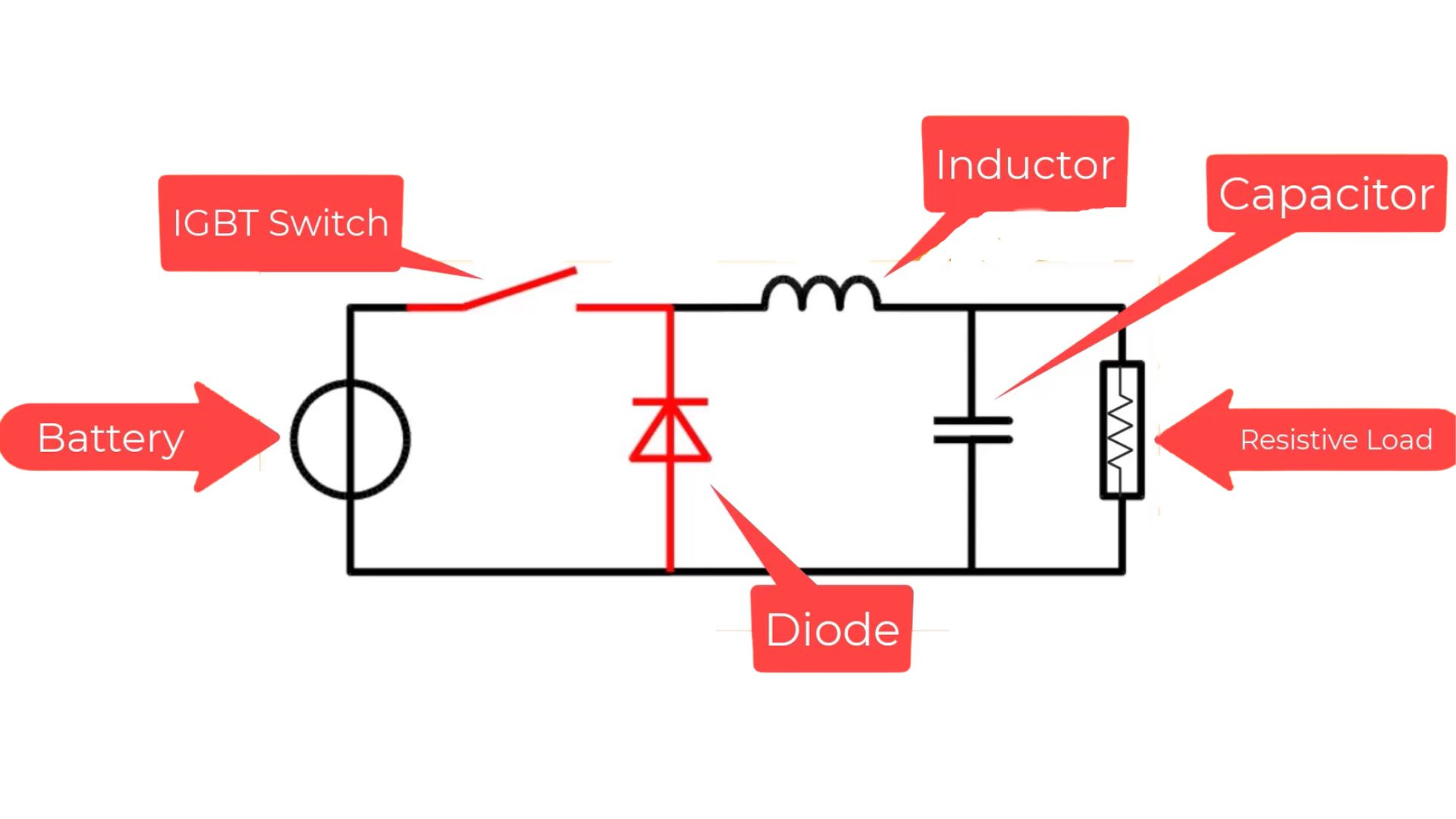The circuit comprises a battery that provides input voltage, a switch for controlling output voltage, two energy storing elements inductor and capacitor for its main circuitry process and a load across which output voltage is measured. It has two states, on and off states. In the on-state, the inductor stores the energy and in the off-state, the capacitor stores and supplies energy where then only a calculated portion of the supply voltage and current is allowed for the load, instead of the relatively bigger peak voltage input source. This is a brief of the process of the buck converter.

## Controlling Measures of Buck Converter

In the power electronics domain or even in real life, controlling aspects are one of the most important aspects always. Things need to be controlled for the safe working of our life or even the circuits before, which might cause some other side effects that might not be good. Here, the value of the output is controlled according to our needs or the controlled voltage value we need across the load for its proper working. There are two types of control measures, i.e., open loop-controlled system and closed loop-controlled system. These two controlling measures will be used in the modelling of the DC-DC buck converter, and the best controlling measure will be found for this circuit.

## Open loop Controlled DC-DC Buck Converter modelling

### What is an Open loop controlling system?

An open loop-controlled system is a control loop system where a group of elements are connected in a sequence to perform a specified function (or task) where the output is controlled without using any feedback and has no influence or effect on the control action of the input signal. There is no chance to correct the transition errors in open loop systems, or there is more chance to occur errors. But still, these controlling systems are widely used in many domains because of the input being free of the output values.

### Modelling of Open-loop Buck converter in MATLAB Simulink

Step into the exciting world of Simulink with MATLAB Helper! Discover the basics of this powerful tool through our complimentary course of Simulink Fundamentals. Ready to show off your Simulink skills? Take our Simulink Fundamentals course quiz and earn a certificate of proficiency from MATLAB Helper. Score 60% or higher and book your certificate now!

The open loop model of buck converter should be made under some considerations where it will be a discrete mode of sample time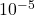(written in Simulink as 1e-5) which makes the model look the same as a buck converter would work in reality.

### Setups before modelling-

• A powergui block is taken from the library browser and select the simulation type discrete and sample time 1e-5.• Click the configuration parameters tool or press Ctrl+e and select the solver type Fixed-step, Solver to discrete (no continuous states) and the Fixed-step size 1e-5
•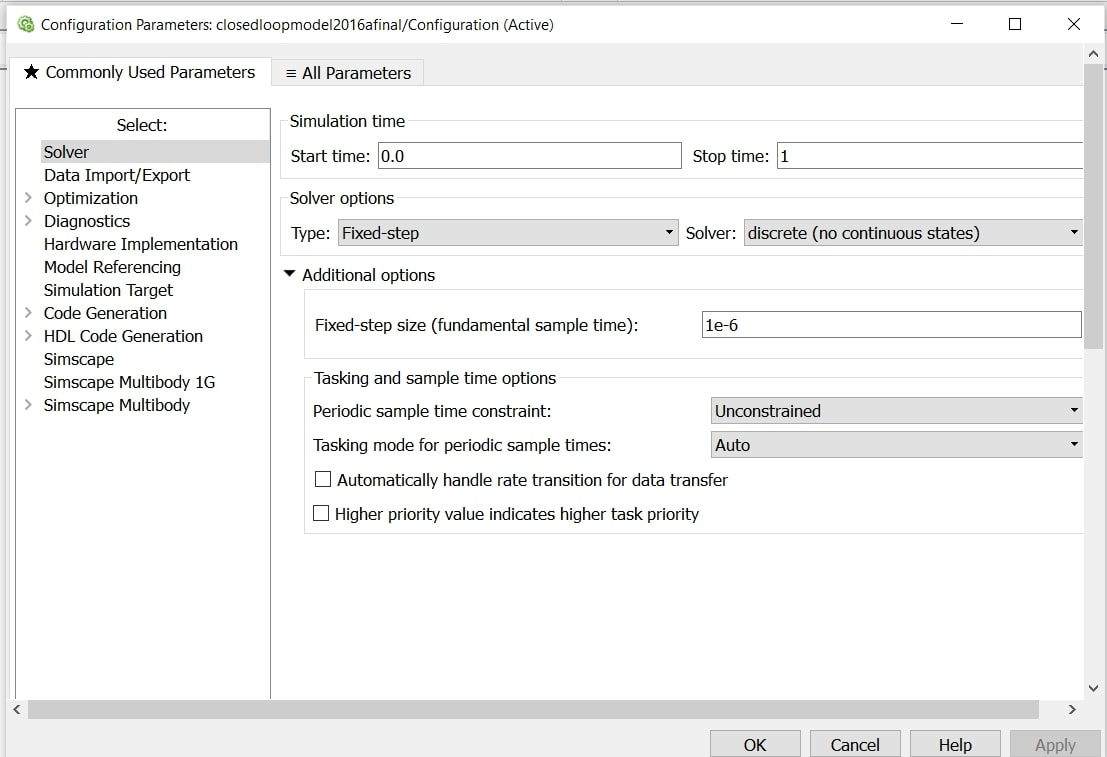### The theory behind selecting the parameters of components: -

There are numerous buck converters made in power electronics of different ratings. Every Converter has a different combination of values of inductor and capacitor according to the ratings required in a particular circuit. Here in this model, a certain combination of inductor and capacitor is taken for which the voltage output is uniform and has fewer ripples. You can also choose a combination of inductor and capacitor for which the voltage output has fewer ripples and is uniform.

The formula of the Buck converter

The output voltage of the buck converter is the input voltage times the duty cycle.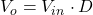Duty Cycle (D)

The duty cycle is the ratio of the signal on time to its total time period.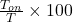In this model, D is taken 0.6 and the RLC branch where,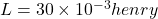farad and R = 1 ohm and input voltage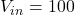Volts

### Modelling: -

• Search the following components in the library and select them for the blank model.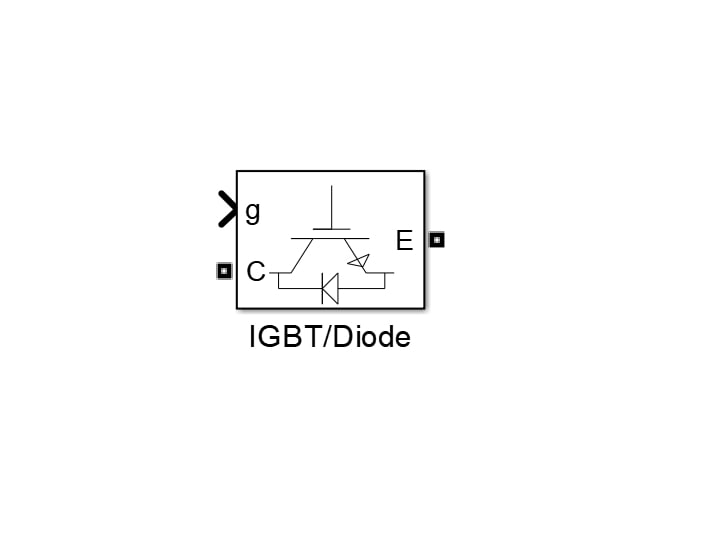Take DC Voltage Source 100Volts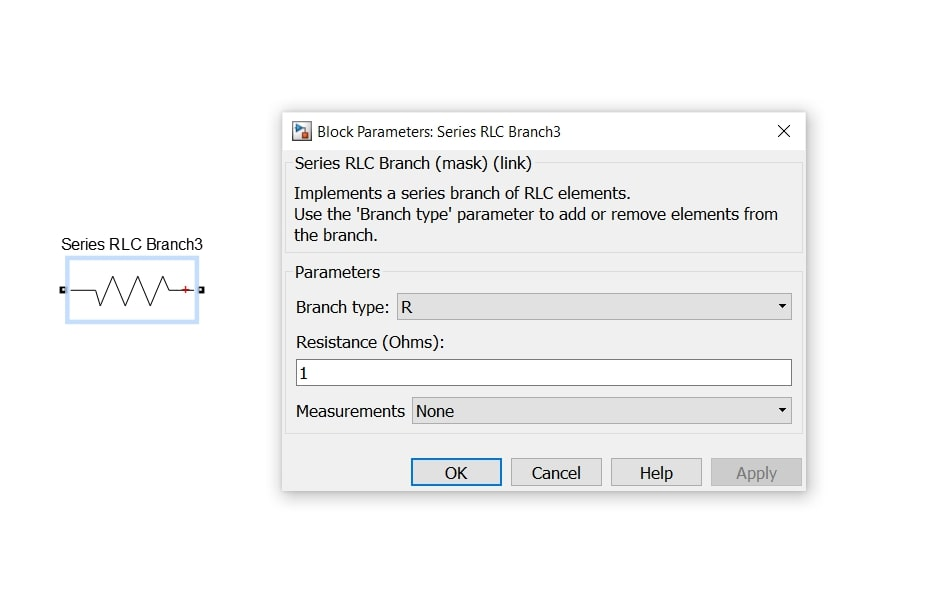Type Resistance 1 ohm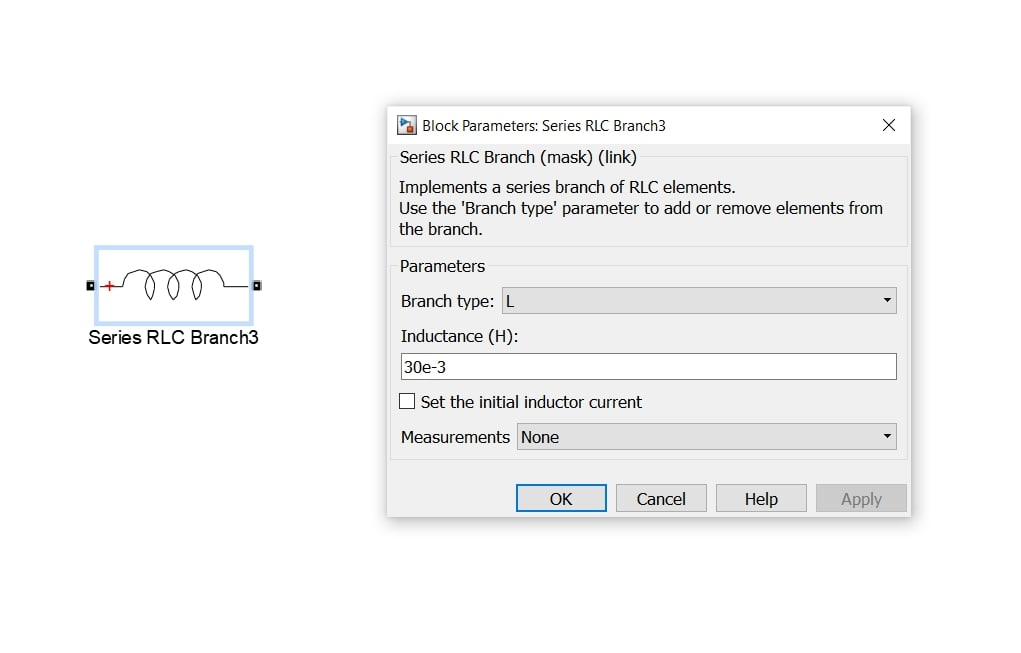Type the inductance 30e-3 i.e.,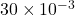henry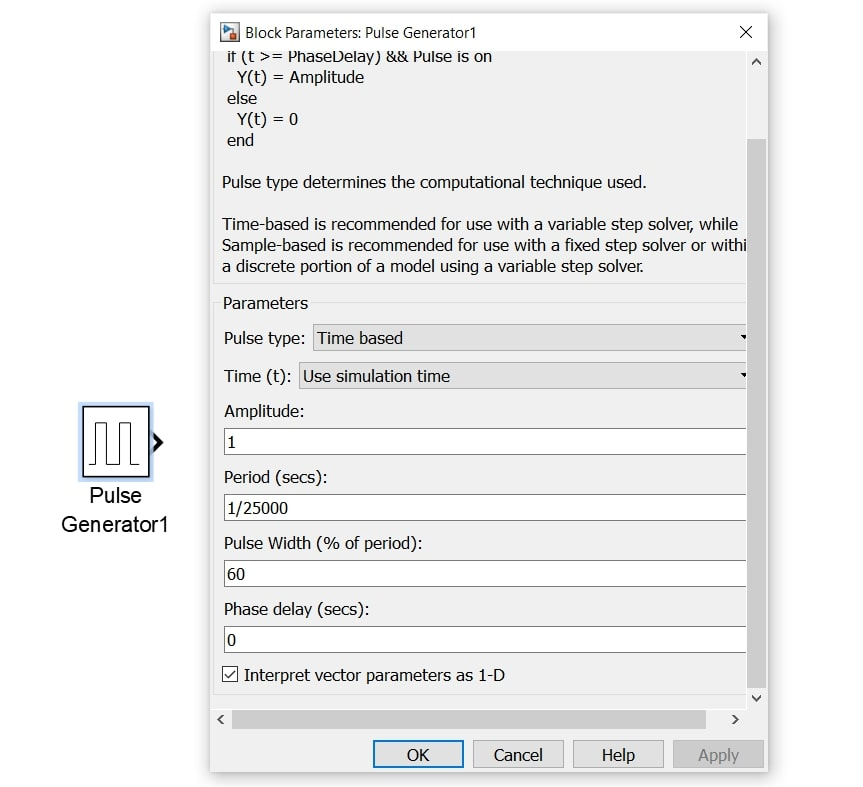Take the period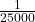and pulse width 60 i.e., D=0.6

• Connect them same as the circuit configuration of buck converter (given above in circuit configuration).
•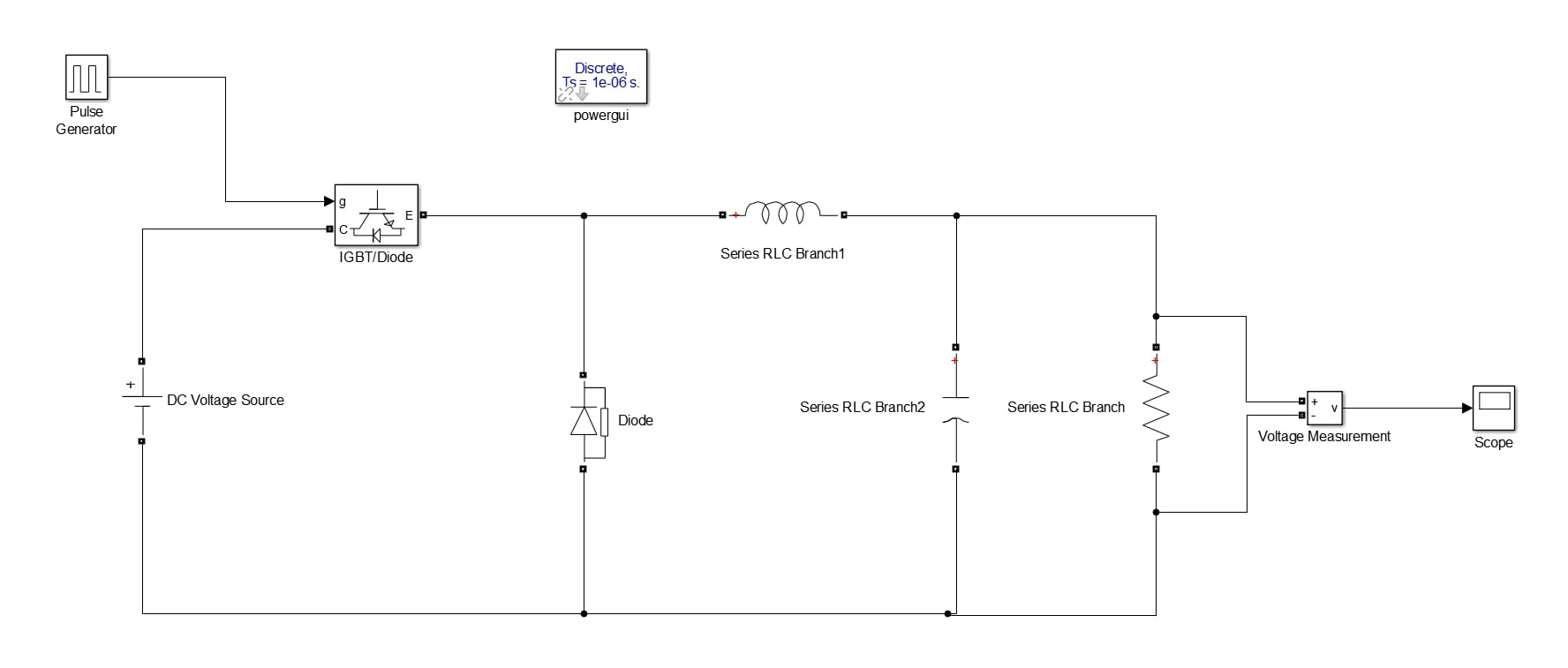• The pulse generator output is connected with the input port of the IGBT input port to control the switch through pulses, and the voltage measurement block is attached parallel to the resistive load to measure the voltage and give the output to the scope to see the results in a graph, and the rest connection is made same as a simple buck converter circuit (please refer to the circuit configuration above).
• Run the model after putting the value of time one second.
•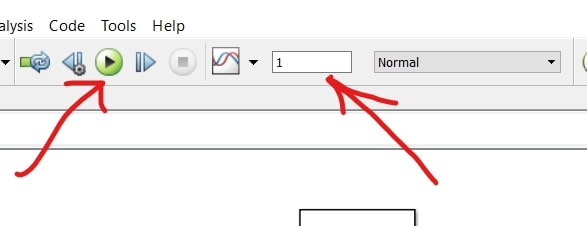• The result after the simulation in the scope is –
•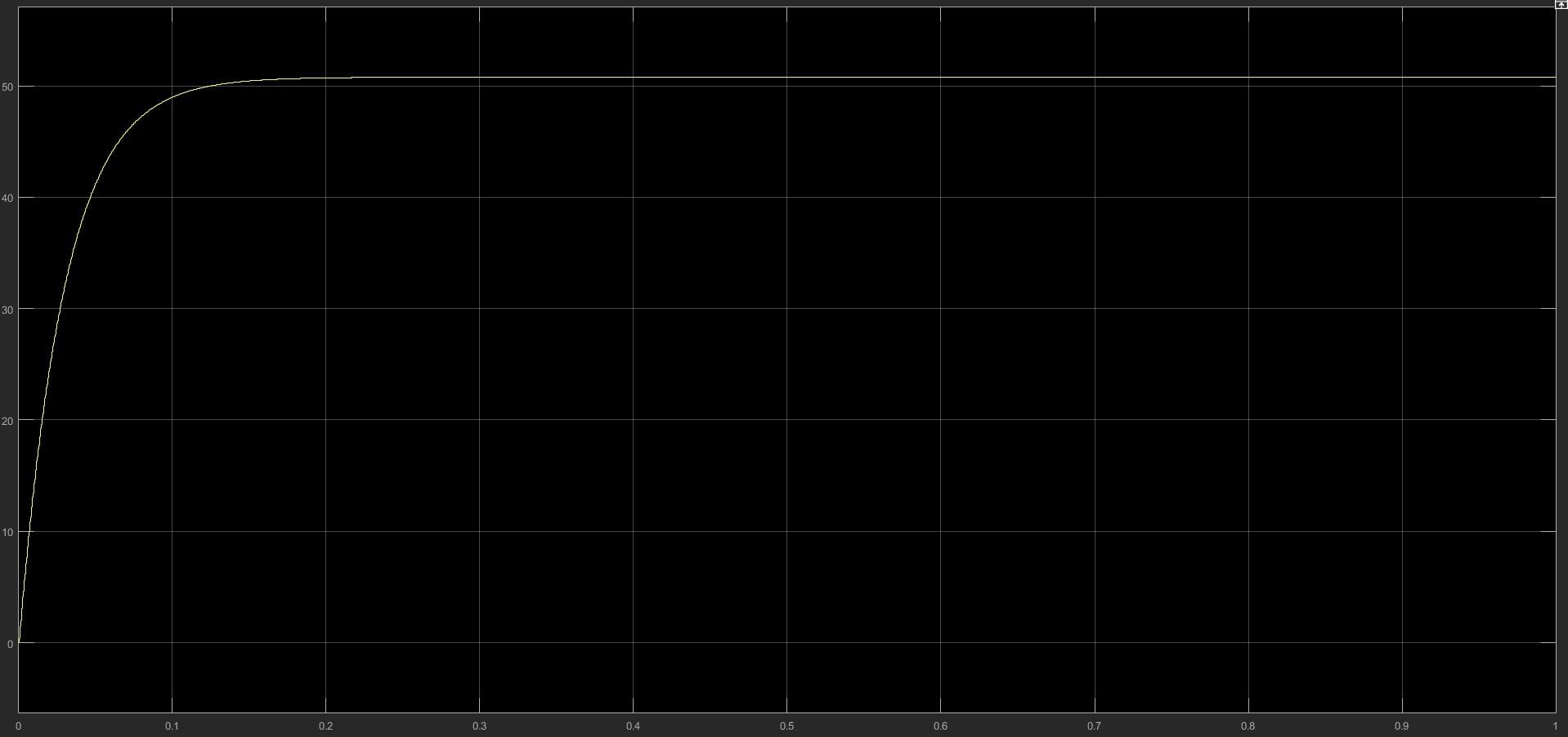After zooming the scope, the graph shows the clear output after setting the voltage to 50; it isn't 50 but near 51 Volts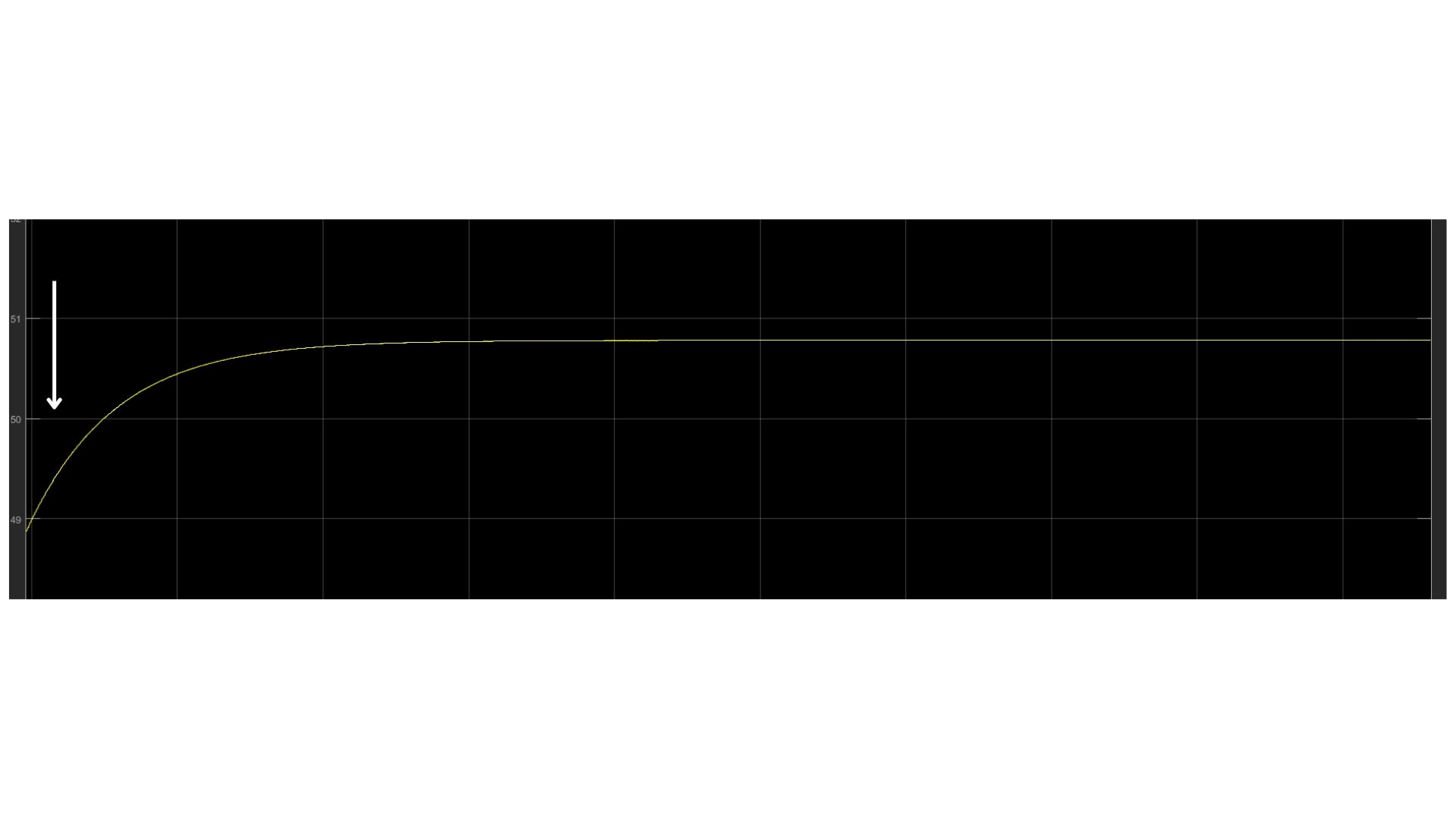• This is the output of an open loop controlled buck converter which, after setting reference voltage, doesn't give voltage perfectly 50, and in the power electronics domain, even a minor fluctuation of voltage is very sensitive to the circuits.
• Let's check out if the closed-loop Converter gives any different results.

Models & Report!

In an open loop buck converter, there is no feedback from output to input, contrary to the closed loop, which has a feedback circuit. Study the comparison of two models with simulation; Developed in MATLAB R2021a with Simulink, Simscape and Simscape Electrical Libraries.

## Closed loop Controlled DC-DC Buck Converter modelling

In the closed loop converter, the IGBT switch is controlled by the pulses generated from the feedback of the output, which is compared with the input and the error or difference is compensated by controllers called PID (Proportional Integral Derivative). Usually, PID is used for very complex circuits; in this model, we will use PI (Proportional Integral) to get a good output.

### What is PID Controller?

PID controller is a controller used in a closed loop feedback system where it takes feedback from the output and compares it with the input, and then compensates or aims to reduce the difference to zero through its proportional, integral and derivative algorithms. Let's see the three terms' characteristics.

#### Proportional: -

• This is the parameter that determines how fast the system responds, for controller term 'Gain' used for proportional.
•• The more the P value, the fast the system will respond and the more sensitive and less stable it will become.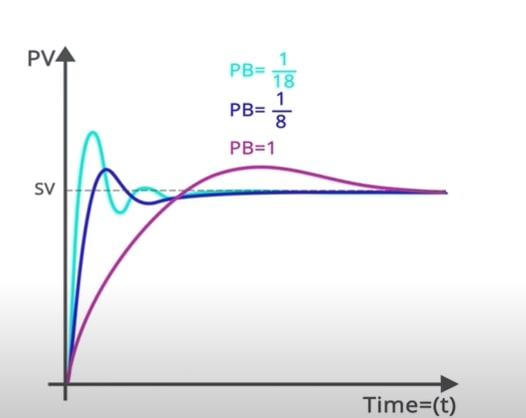#### Integral: -

• The parameter determines how fast, steady-state error is removed.
••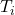is measured in repeats/second, seconds/repeat, repeats/min and mins/repeat
• Smaller minutes per repeat will create larger integral actions, or larger values in repeats per minute measurement will create larger action.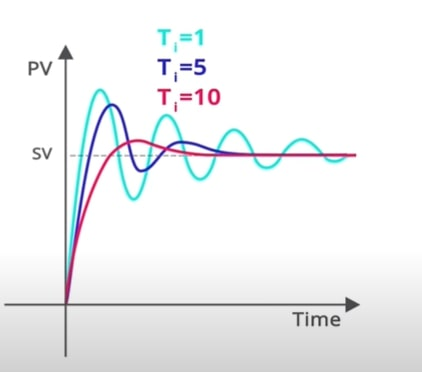#### Derivative: -

• The derivative constant is for predicting change, or the rate of change measured in the process variable or how far in the future you want to predict the rate of change.
• It is the rate of change in the process variable, and the process variable must be a very clean signal; hence no noise within the signal; that's why the derivative is not often used.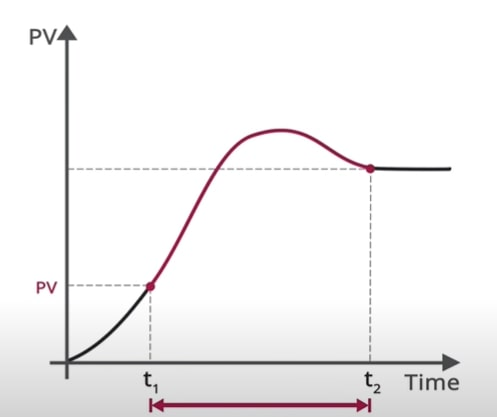### Modelling of Closed loop Buck converter in MATLAB Simulink

#### The theory behind selecting the parameters of components: -

To tune a model through PI, we need to get the transfer function of the model to be tuned. The transfer function of the buck converter is,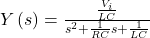After putting the values of L, C and R same as taken in the open loop model, the transfer function looks something like this,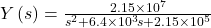#### Modelling: -

Take a transfer function with initial states and put the values the same as calculated.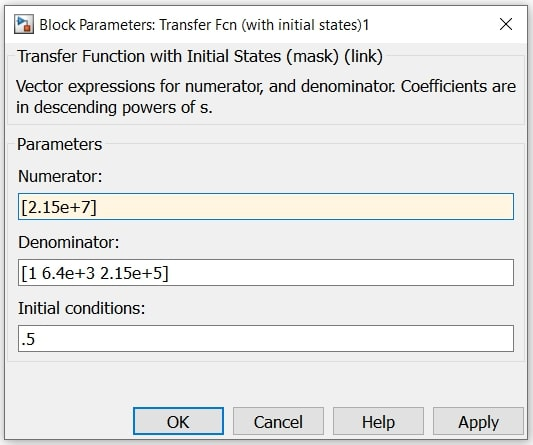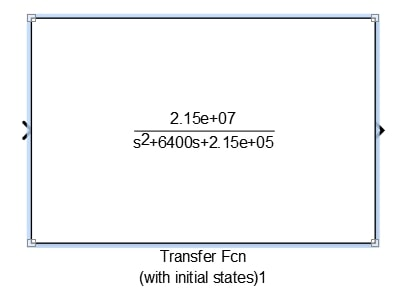• Take the PID controller, select the controller to PI, Time domain to discrete model, sample time to two, then click tune.
•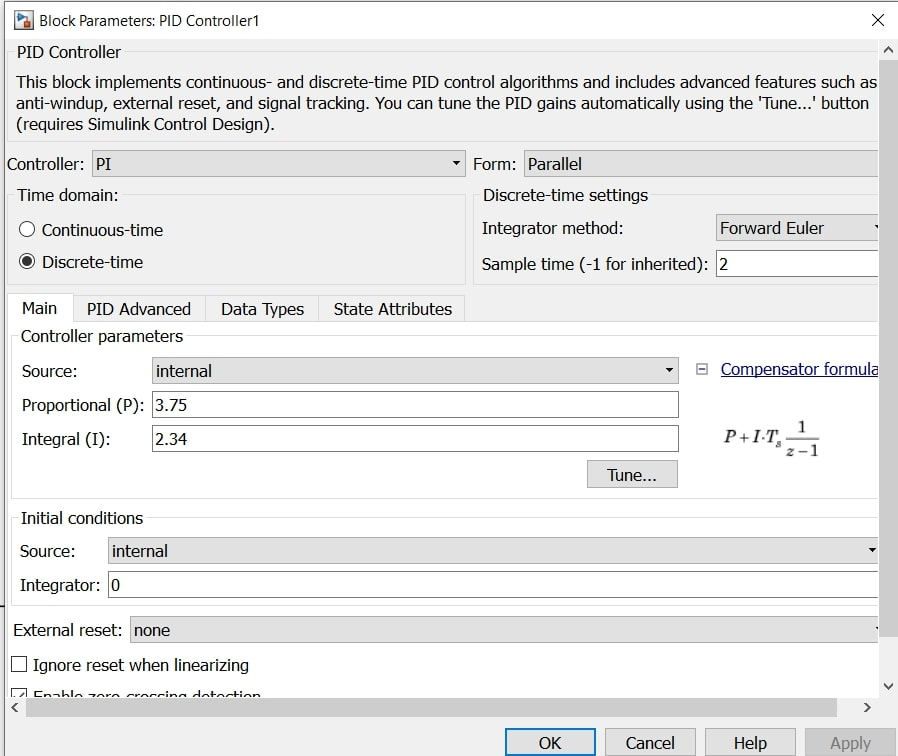• Before tuning, connect the circuit using a scope, sum and constant as shown,
•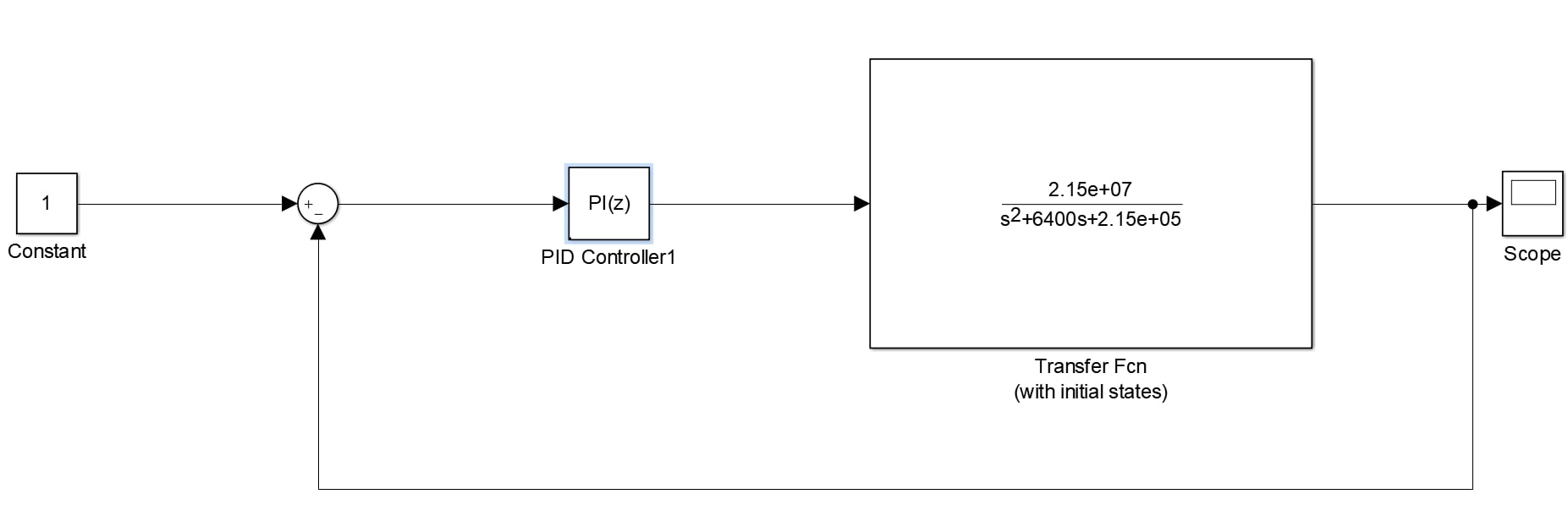• After clicking tune in PI controller, a step plot reference track will open. You can tune the model by adjusting the response time and transient behaviour. On increasing the response, the signal responds fast and improving the transient behaviour to robust the transient in signal reduces. Then click the update block.
•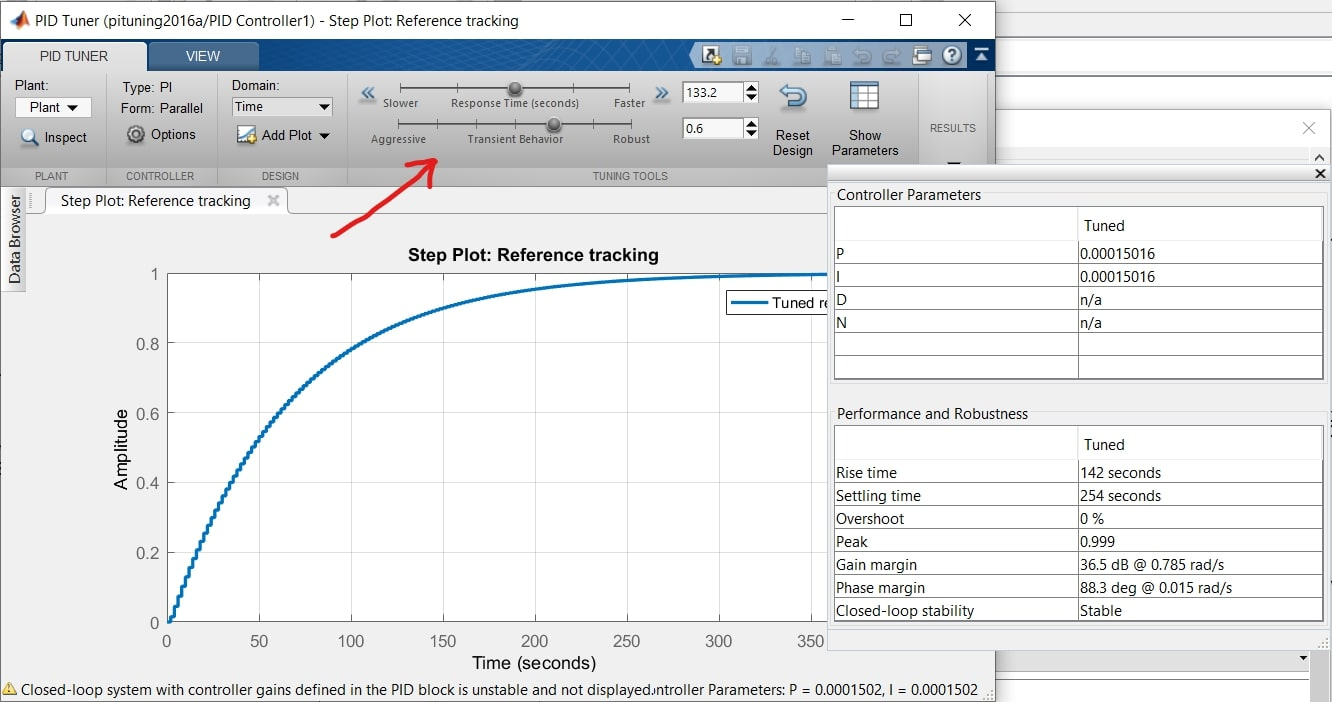• Copy the PI tuned block to the open loop model made and take a constant, sum, add and repeating sequence and connect something like this,
•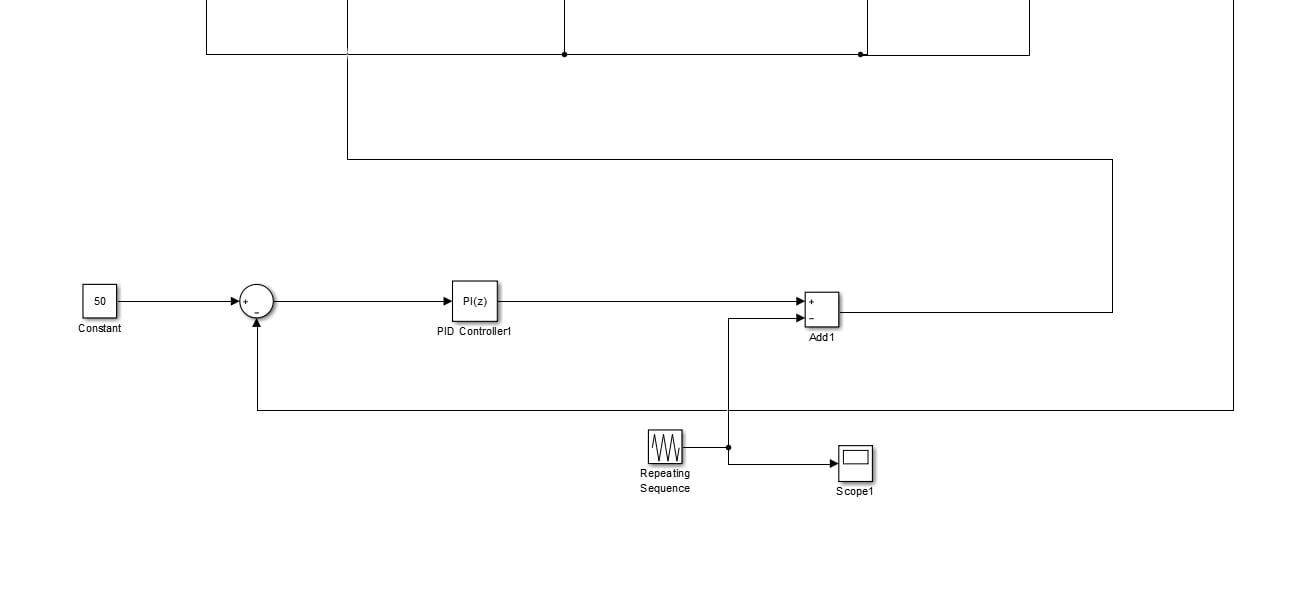• Give feedback of output to negative of sum and set the constant to 50 and make a repeating sequence with such values and generate a pulse that is given to IGBT switch.
•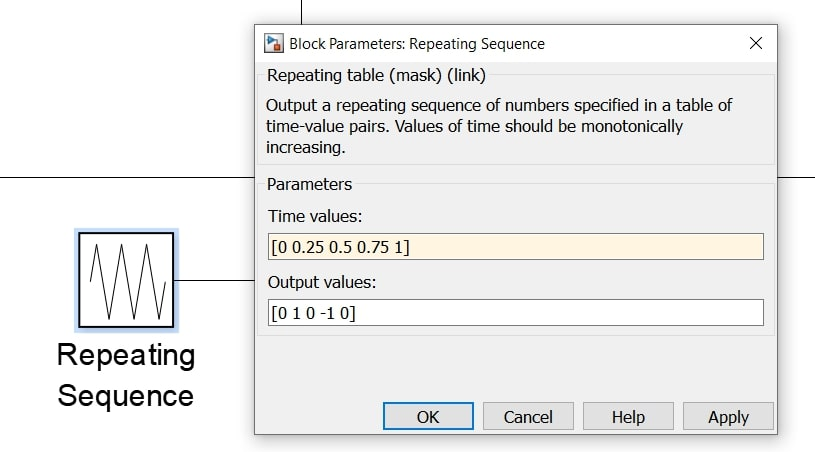• The final design of the model looks something like this.
•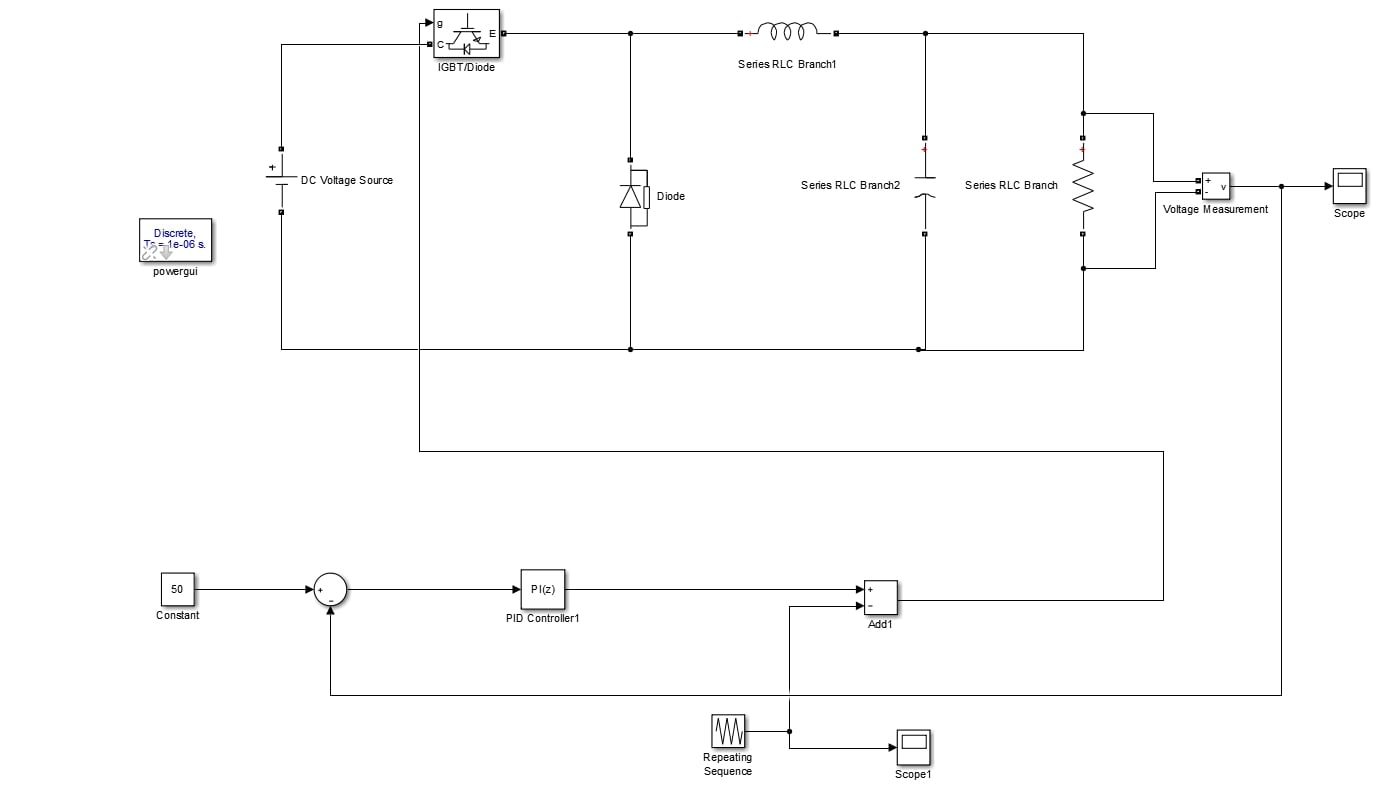• Run the model for one second and check the result in scope.
•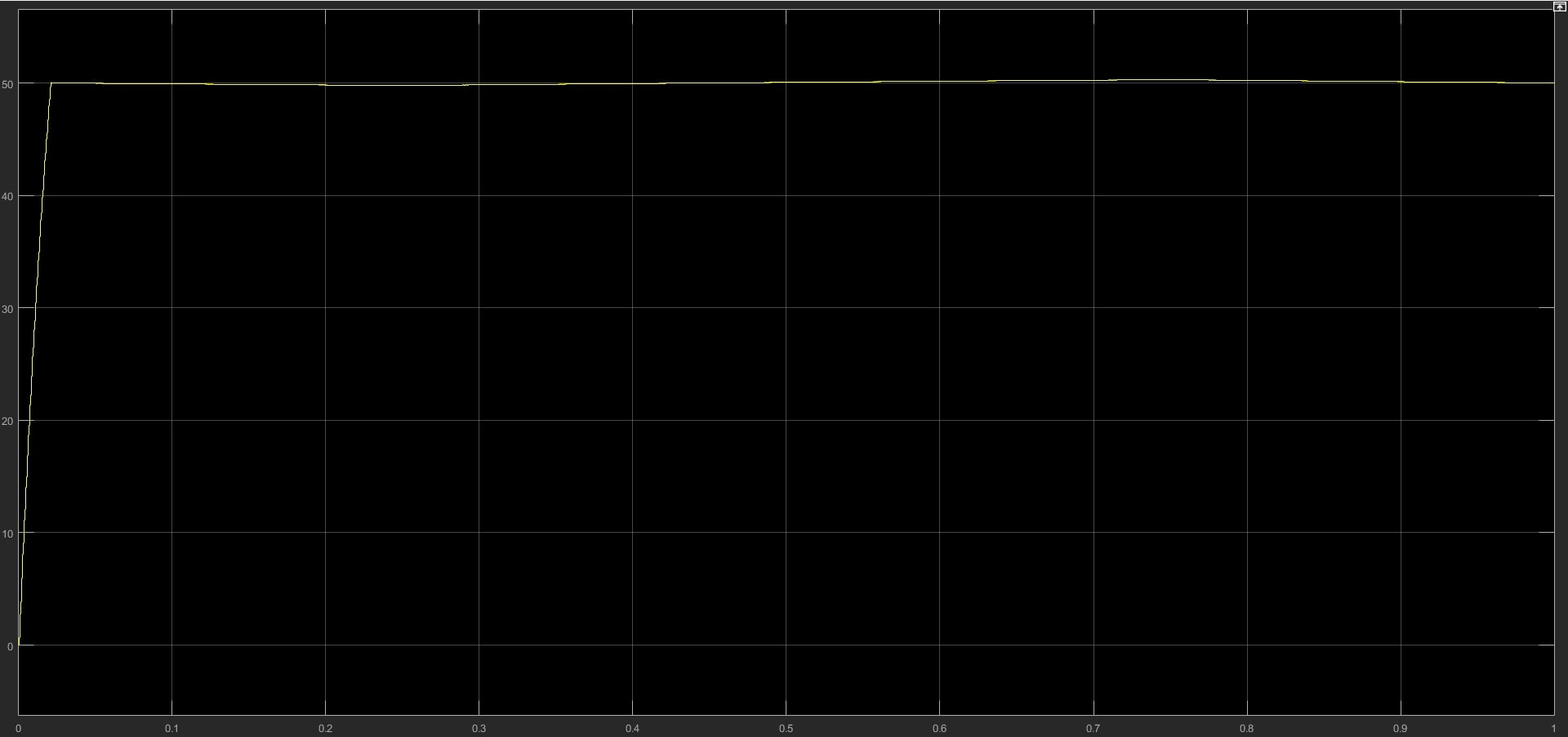• The zoomed output scope shows how precise a closed-loop converter can produce after tuning it perfectly. Still, this model can be tuned more, but for now, this blog is to show the comparison of open loop buck converter output and closed loop buck converter output. Check out the zoomed scope producing voltage nearest to 50 Volts.
•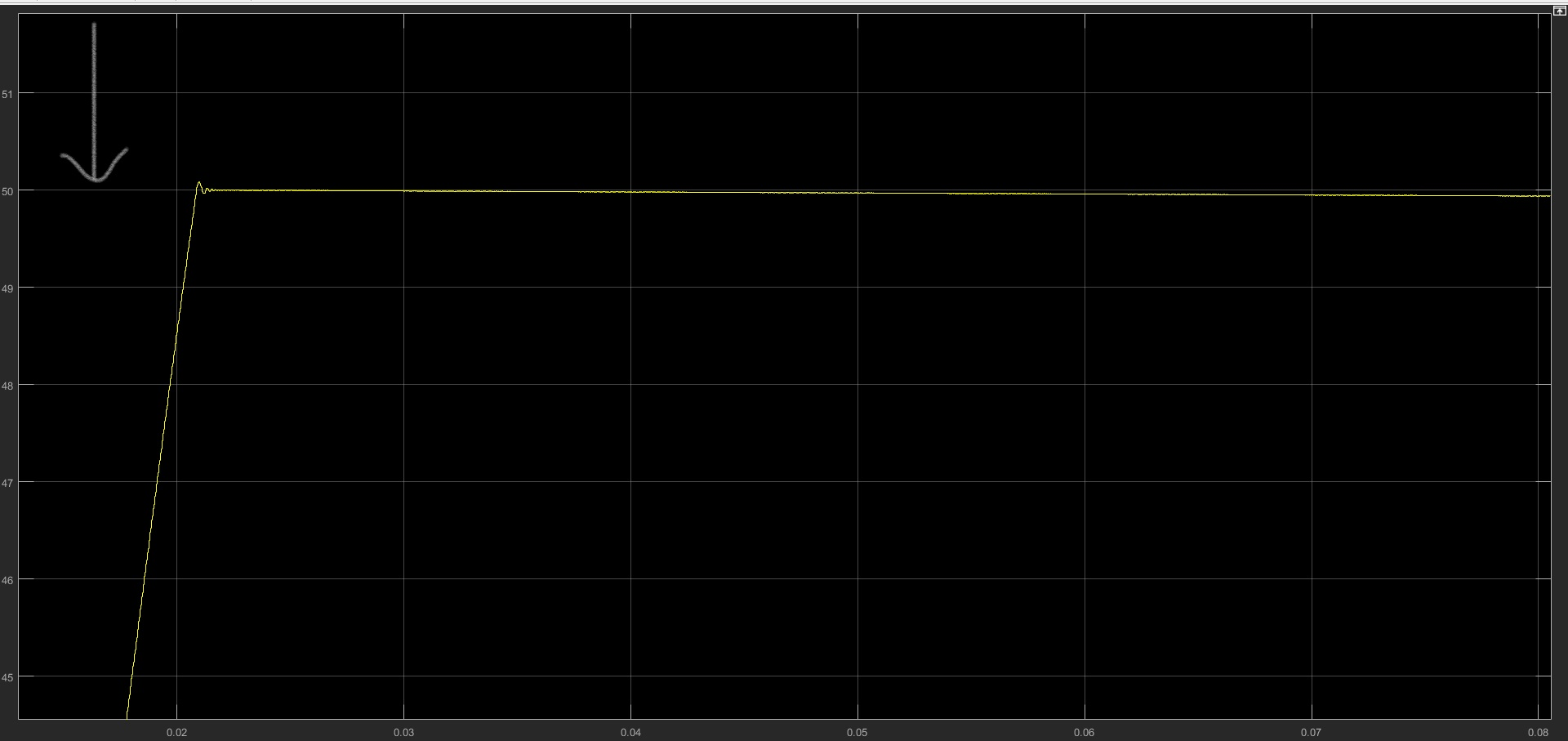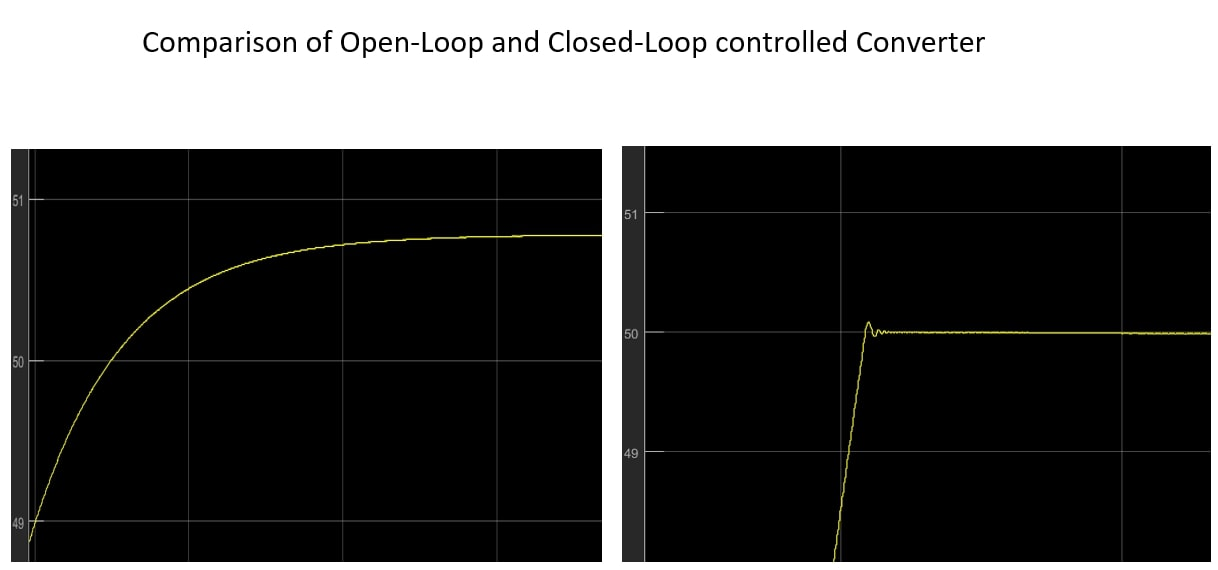## Conclusion

In conclusion, the Buck converter is a widely used DC-DC step-down converter in power electronics, that efficiently converts high input voltages to low output voltages through the use of controlled pulses. The open-loop and closed-loop Buck converters are the two main types of Buck converters, where open-loop converters rely on the inductor to store energy and supply it to the load when the switch is opened and closed-loop converters use feedback to continuously adjust the output voltage.

When comparing the output of the open loop buck converter and closed-loop converter, it is clear that the closed-loop converter gives a better output with precise results due to its feedback system. The closed-loop converter is more accurate and reliable, which makes it an ideal choice for applications that require a steady output voltage.

Ready to take your MATLAB skills to the next level? Look no further! At MATLAB Helper, we've got you covered. From free community support to expert help and training, we've got all the resources you need to become a pro in no time. If you have any questions or queries, don't hesitate to reach out to us. Simply post a comment below or send us an email at [email protected].

And don't forget to connect with us on LinkedIn, Facebook, and Subscribe to our YouTube Channel! We're always sharing helpful tips and updates, so you can stay up-to-date on everything related to MATLAB. Plus, if you spot any bugs or errors on our website, just let us know and we'll make sure to fix it ASAP.

Ready to get started? Book your expert help with Research Assistance plan today and get personalized assistance tailored to your needs. Or, if you're looking for more comprehensive training, join one of our training modules and get hands-on experience with the latest techniques and technologies. The choice is yours – start learning and growing with MATLAB Helper today!

Education is our future. MATLAB is our feature. Happy MATLABing!

0 shares

Tags

buck converter, closed loop, dc dc buck converter, open loop, open loop vs closed loop

I am working as a MATLAB Developer Intern at MATLAB Helpers.

## Runge-Kutta method in MATLAB

Runge-Kutta method in MATLAB

## Lidar Point Cloud Data Processing

Lidar Point Cloud Data Processing
•Zawar Kanju says:

I am very impressed with your post because this post is very beneficial for me and provide a new knowledge…

•Aniket says:

Thanks. This Helped 🙂

•Paul kihara says:

Very educative article

•icecoldsoder says:

Where did you get the transfer function of the buck converter from?

Yleft ( s right )= frac{frac{V_{i}}{LC}}{s^{2}+frac{1}{RC}s+frac{1}{LC}}

#### MATLAB Helper ®

Use Website Chat or WhatsApp at +91-8104622179